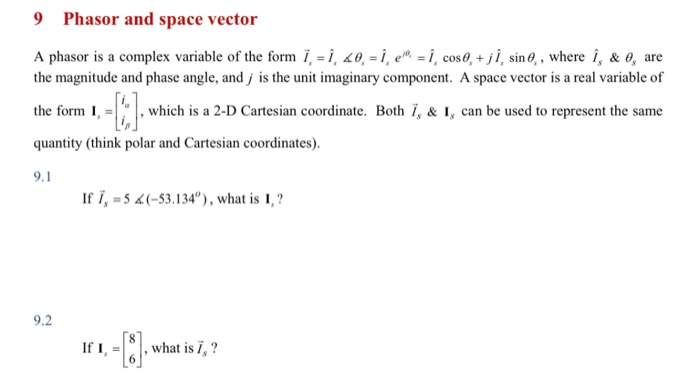# 9 Phasor and space vector A phasor is a complex variable of the form i, = Î, 20, = Î , e™ = i, cos 0, + j Î, sin, , where î, & o, are the magnitude and phase angle, and j is the unit imaginary component. A space vector is a real variable of the form I, = , which is a 2-D Cartesian coordinate. Both Ï, & I, can be used to represent the same quantity (think polar and Cartesian coordinates). 9.1 If i, = 5 4(-53.134"), what is I, ? 9.2 If I, what is 7, ?

Questionhelp_outlineImage Transcriptionclose9 Phasor and space vector A phasor is a complex variable of the form i, = Î, 20, = Î , e™ = i, cos 0, + j Î, sin, , where î, & o, are the magnitude and phase angle, and j is the unit imaginary component. A space vector is a real variable of the form I, = , which is a 2-D Cartesian coordinate. Both Ï, & I, can be used to represent the same quantity (think polar and Cartesian coordinates). 9.1 If i, = 5 4(-53.134"), what is I, ? 9.2 If I, what is 7, ? fullscreen

### Want to see this answer and more?

Experts are waiting 24/7 to provide step-by-step solutions in as fast as 30 minutes!*

*Response times may vary by subject and question complexity. Median response time is 34 minutes for paid subscribers and may be longer for promotional offers.
Tagged in
Engineering
Electrical Engineering

### Circuit Theory